Next: 8. Summary and Conclusions Up: 7. Case Studies Previous: 7.3 6T SRAM Cell

Subsections

# 7.4 Ring OscillatorIn this section a five stage CMOS ring oscillator, as depicted in Figure 7.22, is investigated with regard to NBTI degradation. A ring oscillator comprises of an odd number of CMOS inverters. The output of each inverter is used as input for the next one. The last output is fed back to the first inverter. Because of the delay time of each stage the whole circuit spontaneously starts oscillating at a certain frequency. The frequencydepends on the number of stages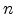and the delay time of the inverters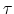as follows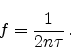(7.3)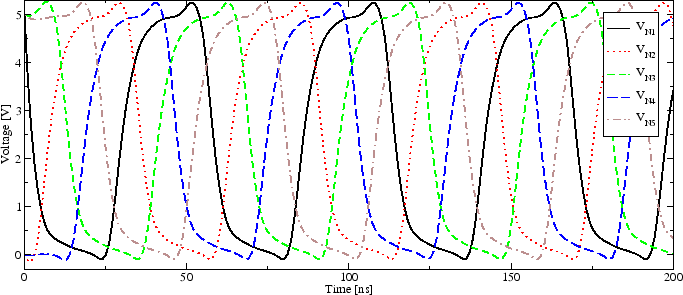In the simulation the initial condition for the node voltages-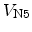has to be defined. In the first time step the voltages at node N1, N3, and N4 are forced to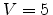V and the others toV. The voltages quickly reach their oscillation voltages.

Figure 7.23 shows the transient oscillation of the inverter. This non-degraded circuit oscillates with a frequency of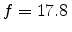MHz. Using (7.3) the delay time of the inverters calculates asns.

## 7.4.1 Frequency Degradation

Negative bias temperature instability mainly affects the p-channel transistors in the inverter circuits. Their level of degradation is approximately equal because of the identical stress conditions in the oscillating circuit.

NBTI leads to an increased absolute threshold voltage,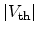. This, in turn, reduces the gate overdrive required to turn on the p-channel transistors. When turning the transistor off, on the other hand side, the overdrive is increased and this process is therefore performed faster, as shown in Section 7.2.3. Still, as the lower turn-on speed dominates, the inverter's delay time increases,,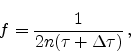(7.4)

reducing the oscillation frequency.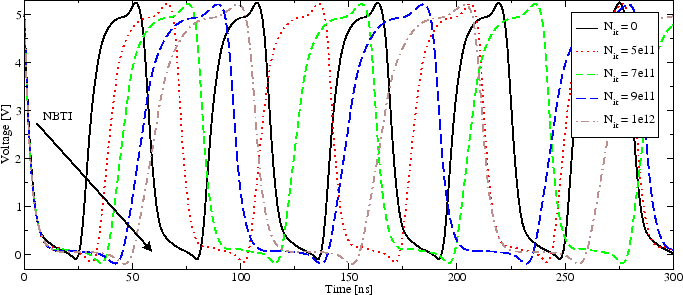Figure 7.24 depicts the oscillation voltage of the unstressed circuit and after degradation due to NBTI. A clear reduction of the oscillation frequency can be observed. With a very large interface degradation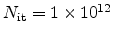the oscillation frequency is reduced fromMHz to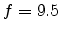MHz. The delay time of the five inverters is increased by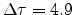ns.

Figure 7.25: Frequency degradation versus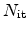. As predicted by (7.4) the frequency of the ring oscillator is drastically reduced with increasing NBTI degradation.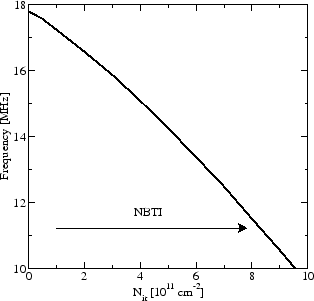Linear scale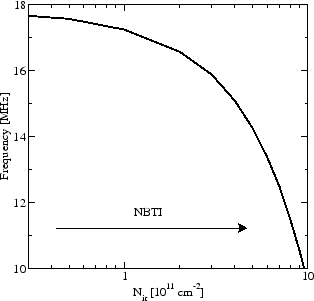Logarithmic scale

The evolution of the frequency with NBTI induced interface trap generation can be seen in Figure 7.25. Increasing degradation leads to a reduced oscillation frequency of the ring oscillator, as predicted by (7.4).

## 7.4.2 Transient Degradation

To evaluate the effect of long time NBT stress on the ring oscillator's frequency degradation a transient NBTI simulation of a single p-channel MOSFET has been performed. Figure 7.26 shows the resulting interface trap densityat constant voltage and constant temperature stress for 10 years using the new model. When assuming a frequency degradation of 5% to be within the circuits design rules, Figure 7.26(a) shows very well that at temperatures above 100^C and with a stress voltage ofV the degradation exceeds this limit within 10 years (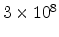s). At regular operating voltage with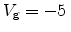V the degradation limit is not reached.

It has to be considered, though, that for these simulations constant stress was assumed. As shown in Chapter 6 NBTI has a recovery effect when the stress conditions are removed. Therefore, Figure 7.27 gives the resulting degradation at periodic stress with 1Hz oscillation frequency for 1 hour. It is not possible to simulate the whole 10 years of lifetime, as for each second at least two simulations have to be performed, but the trend is clear. The overall degradation is reduced for periodic stress voltages and stays below the degradation at permanent stress conditions. This enhances the lifetime as defined above considerably.

The same simulations have been performed using the standard reaction-diffusion model (Figure 7.28 and 7.29). The estimated degradation after 10 years stress is clearly different and even lies beyond the 5% border for all temperatures and voltages. This result emphasizes how important the use of a correct model and the right model parameters is for long time predictions.

Figure 7.26: p-channel MOSFET degradation simulation at constant NBT stress for 10 years using the new model. A 5 % frequency degradation of the ring oscillator is observed at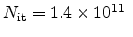cm. Only at (a) 25 V gate stress and more than 100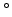C the degradation threshold is reached within 10 years. At regular operating voltage (b) the frequency degradation stays within 5 % in 10 years.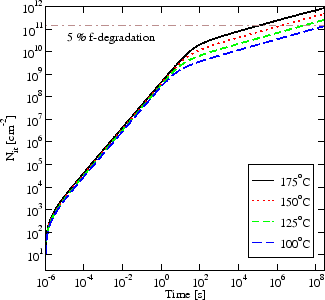VV

Figure 7.27: p-channel MOSFET degradation simulation at dynamic NBT stress for 1 hour using the new model. The stress voltage oscillates with 1 Hz and a duty cycle of 50 % (dashed lines) and with constant stress (solid lines) have been used. The level of degradation with oscillating stress is always below constant stress and increases the lifetime.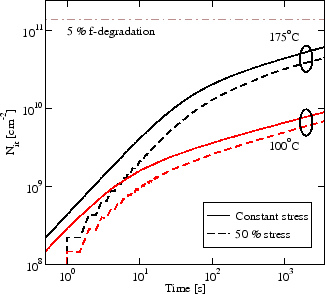V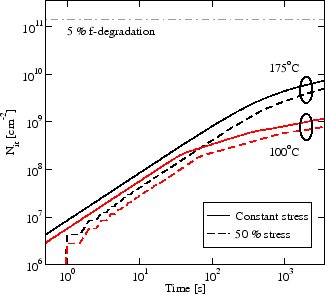V

Figure 7.28: p-channel MOSFET degradation simulation at constant NBT stress for 10 years using the standard reaction-diffusion model. The simulation shows how crucial the use of the correct model is for long time predictions. The R-D model predicts that the ring oscillator would hardly reach the 5 % degradation within 10 years even at the harshest stress conditions.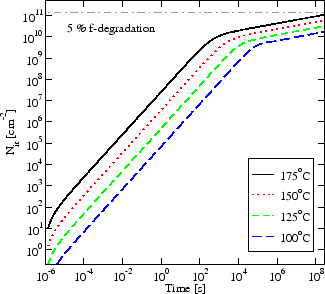V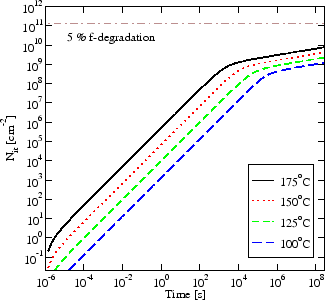V

Figure 7.29: p-channel MOSFET degradation simulation at dynamic NBT stress for 1 hour using the reaction-diffusion model.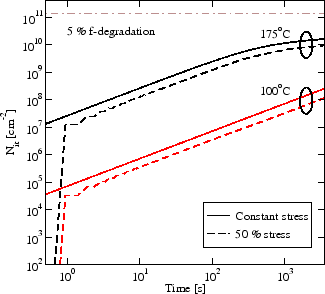V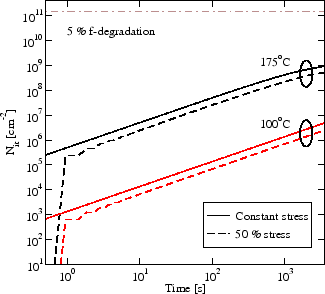VNext: 8. Summary and Conclusions Up: 7. Case Studies Previous: 7.3 6T SRAM Cell

R. Entner: Modeling and Simulation of Negative Bias Temperature Instability